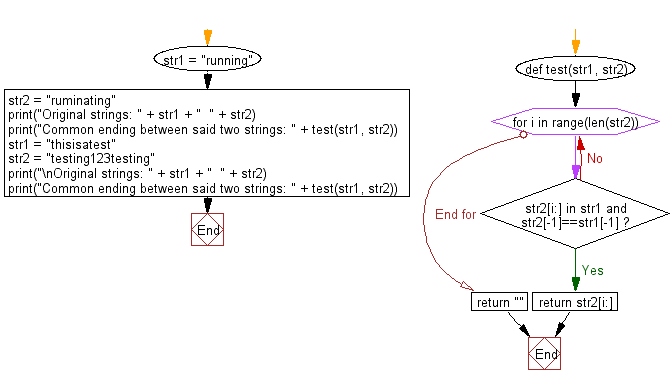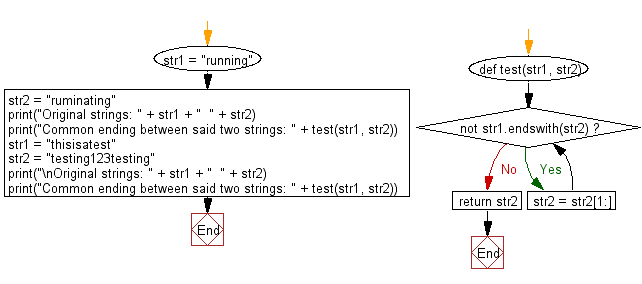﻿ Python: Find the longest common ending between two given strings - w3resource

# Python: Find the longest common ending between two given strings

## Python Basic - 1: Exercise-137 with Solution

Write a Python program to find the longest common ending between two given strings.

Sample Solution-1:

Python Code:

``````def test(str1, str2):
for i in range(len(str2)):
while str2[i:] in str1 and str2[-1]==str1[-1]:
return str2[i:]
return ""

str1 = "running";
str2 = "ruminating";
print("Original strings: " + str1 + "  " + str2);
print("Common ending between said two strings: " + test(str1, str2));
str1 = "thisisatest";
str2 = "testing123testing";
print("\nOriginal strings: " + str1 + "  " + str2);
print("Common ending between said two strings: " + test(str1, str2));
``````

Sample Output:

```Original strings: running  ruminating
Common ending between said two strings: ing

Original strings: thisisatest  testing123testing
Common ending between said two strings:
```

Flowchart:Sample Solution-2:

Python Code:

``````def test(str1, str2):
while not str1.endswith(str2):
str2 = str2[1:]
return str2
str1 = "running";
str2 = "ruminating";
print("Original strings: " + str1 + "  " + str2);
print("Common ending between said two strings: " + test(str1, str2));
str1 = "thisisatest";
str2 = "testing123testing";
print("\nOriginal strings: " + str1 + "  " + str2);
print("Common ending between said two strings: " + test(str1, str2));
``````

Sample Output:

```Original strings: running  ruminating
Common ending between said two strings: ing

Original strings: thisisatest  testing123testing
Common ending between said two strings:
```

Flowchart:## Visualize Python code execution:

The following tool visualize what the computer is doing step-by-step as it executes the said program:

Python Code Editor:

Have another way to solve this solution? Contribute your code (and comments) through Disqus.

What is the difficulty level of this exercise?

Test your Programming skills with w3resource's quiz.

﻿